﻿ 基于泰勒展开的线性多步法构造方法的研究 A Study on Construction for Linear Multi-Step Methods Based on Taylor Expansion

Advances in Applied Mathematics
Vol.04 No.04(2015), Article ID:16357,7 pages
10.12677/AAM.2015.44042

A Study on Construction for Linear Multi-Step Methods Based on Taylor Expansion

Zhiyuan Huang1, Zhijun Hu2, Cheng Wang3

1Guangzhou University, Guangzhou Guangdong

2Guangxi Normal University, Guilin Guangxi

3Huizhou University, Huizhou Guangdong

Received: Oct. 30th, 2015; accepted: Nov. 13th, 2015; published: Nov. 18th, 2015

Copyright © 2015 by authors and Hans Publishers Inc.

This work is licensed under the Creative Commons Attribution International License (CC BY).

http://creativecommons.org/licenses/by/4.0/ABSTRACT

Following the previous literature on multi-step formulae for initial value problems of ODEs (ordinary differential equations), we study the construction for linear multi-step methods based on Taylor expansion in this paper. We try the weighted average method and derive a new formula. Then we check this new method in an example, and compare the original two formulae and constructed new formula. Via such numerical experiment, this method is reliable. Some constructed new formulae can have relatively high stability and small error while solving ODEs initial value problems.

Keywords:Initial Value Problems, Linear Multi-Step Methods, Stability, Weighted Average Method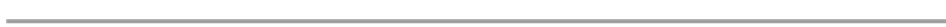1广州大学，广东 广州

2广西师范大学，广西 桂林

3惠州学院，广东 惠州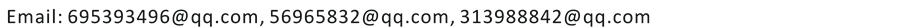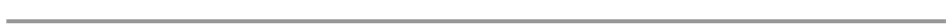1. 引言

2. 用加权平均方法构造新的线性多步法公式

2.1. 线性多步法的局部截断误差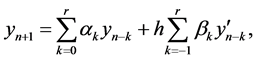(2.1.1)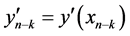，由Taylor展开，有(2.1.2)(2.1.3)(2.1.4)(2.1.5)

2.2. 用加权平均方法构造新公式的基本思想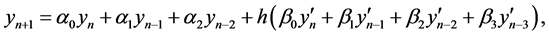(2.2.1)(2.2.2)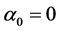,,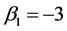,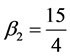,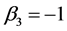.(2.2.3)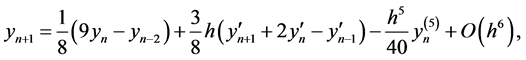(2.2.4)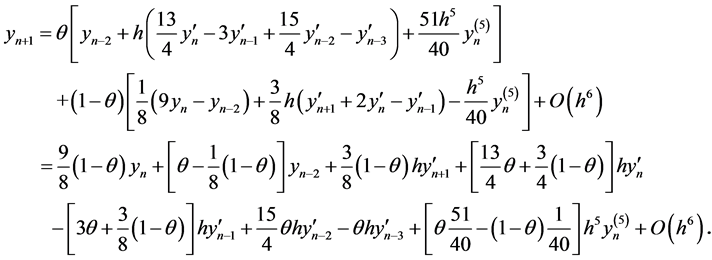(2.2.5)(2.2.6)(2.2.7)(2.2.8)

2.3. 数值实验(2.3.1)

(初值问题(2.3.1)有精确解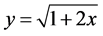。)Figure 1. Curve: Image result of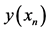and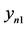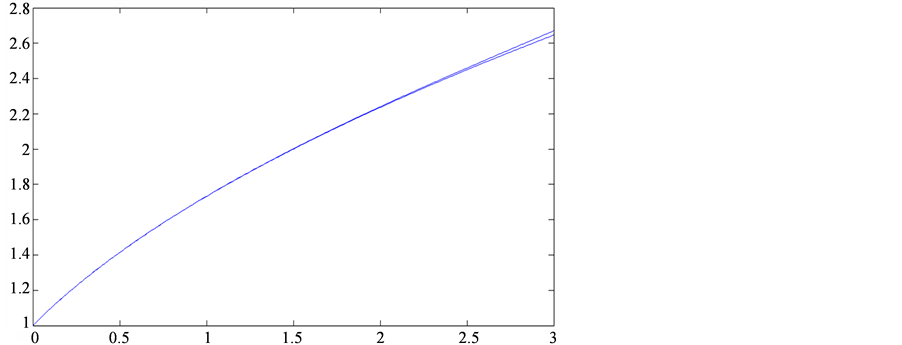Figure 2. Curve: Image result ofand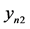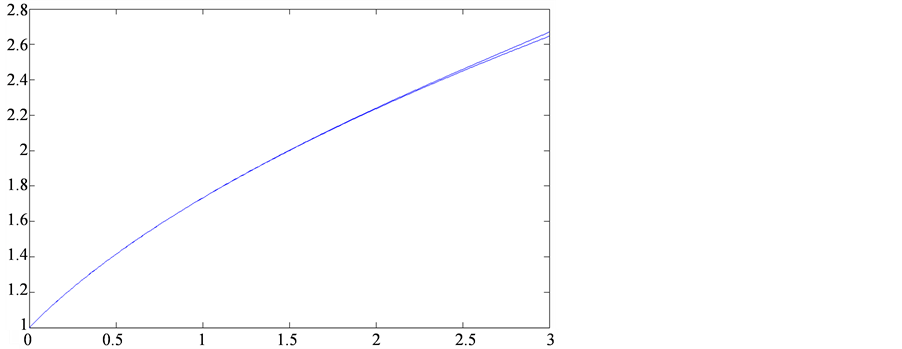Figure 3. Curve: Image result ofand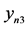3. 结论

A Study on Construction for Linear Multi-Step Methods Based on Taylor Expansion[J]. 应用数学进展, 2015, 04(04): 336-342. http://dx.doi.org/10.12677/AAM.2015.44042

1. 1. Atkinson, K.E., 著. 数值分析引论[M]. 匡蛟勋, 译. 上海: 上海科学技术出版社, 1986.

2. 2. 李庆扬, 关治, 白峰杉. 数值计算原理[M]. 北京: 清华大学出版社, 2000.

3. 3. 关治, 陆金甫. 数值分析基础[M]. 北京: 高等教育出版社, 1998.

4. 4. 李庆扬, 王能超, 易大义. 数值分析[M]. 第4版. 武汉: 华中科技大学出版社, 2006.

5. 5. Henrici, P. (1962) Discrete Variable Methods in Ordinary Differential Equations. Wiley, New York.

6. 6. 李大侃, 编. 常微分方程数值解[M]. 杭州: 浙江大学出版社, 1994.

7. 7. 李庆扬. 常微分方程数值解法[M]. 北京: 高等教育出版社, 1992.

8. 8. 李荣华, 冯果忱. 微分方程的数值解法[M]. 第3版. 北京: 高等教育出版社, 1996.

9. 9. Dahlquist, G. (1963) A Special Stability Problem for Linear Multistep Methods. BIT, 3, 27-43.

10. 10. Butcher, J.C. (1975) A Stability Property of Implicity Runge-Kutta Methods. BIT, 15, 358-361.

11. 11. 刘丹. 常微分方程数值解的长时间性态[D]: [硕士论文]. 哈尔滨: 黑龙江大学, 2004.

12. 12. 吕万金. 一类常微分方程长时间数值计算稳定性分析[J]. 黑龙江大学自然科学学报, 2000, 17(4): 4-6.